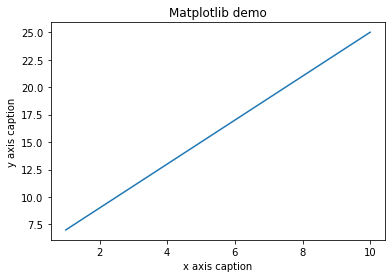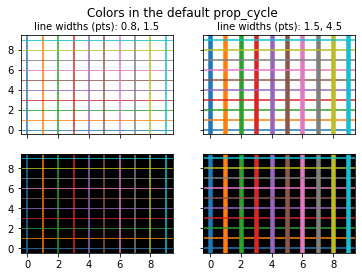# Stub 3 (Machine Learning Examples)

$$\newcommand{\vecs}{\overset { \rightharpoonup} {\mathbf{#1}} }$$ $$\newcommand{\vecd}{\overset{-\!-\!\rightharpoonup}{\vphantom{a}\smash {#1}}}$$$$\newcommand{\id}{\mathrm{id}}$$ $$\newcommand{\Span}{\mathrm{span}}$$ $$\newcommand{\kernel}{\mathrm{null}\,}$$ $$\newcommand{\range}{\mathrm{range}\,}$$ $$\newcommand{\RealPart}{\mathrm{Re}}$$ $$\newcommand{\ImaginaryPart}{\mathrm{Im}}$$ $$\newcommand{\Argument}{\mathrm{Arg}}$$ $$\newcommand{\norm}{\| #1 \|}$$ $$\newcommand{\inner}{\langle #1, #2 \rangle}$$ $$\newcommand{\Span}{\mathrm{span}}$$ $$\newcommand{\id}{\mathrm{id}}$$ $$\newcommand{\Span}{\mathrm{span}}$$ $$\newcommand{\kernel}{\mathrm{null}\,}$$ $$\newcommand{\range}{\mathrm{range}\,}$$ $$\newcommand{\RealPart}{\mathrm{Re}}$$ $$\newcommand{\ImaginaryPart}{\mathrm{Im}}$$ $$\newcommand{\Argument}{\mathrm{Arg}}$$ $$\newcommand{\norm}{\| #1 \|}$$ $$\newcommand{\inner}{\langle #1, #2 \rangle}$$ $$\newcommand{\Span}{\mathrm{span}}$$$$\newcommand{\AA}{\unicode[.8,0]{x212B}}$$

## NumPy

There are many python libraries that are useful for machine learning. On of the most foundational libraries used in NumPy. Through a NumPy array it is easy to represent 2-D datasets as a matrix. Columns in the matrix can represent features, and rows can be samples, or vice versa. Here is an easy way for making a 2x3 NumPy array containing zeros.

import numpy as np
np.zeros((2, 3))
array([[0., 0., 0.], [0., 0., 0.]])

Notice that the library needs to be imported in order to be implemented. Furthermore, NumPy represents matrices in a series of lists. If there are 2 rows, there will be 2 lists. If there are 3 columns, then there are 3 elements in each list. If you already have a list of values that you want to make into a NumPy array, then that is also possible.

import numpy as np
np.array([[1,2,3,4], [5,6,7,8], [9,10,11,12], [13,14,15,16]])
array([[ 1, 2, 3, 4], [ 5, 6, 7, 8], [ 9, 10, 11, 12], [13, 14, 15, 16]])

## MatPlotLib

A great part about NumPy arrays is that they can be used with MatPlotLib to plot the data points in the matrix.

import numpy as np
from matplotlib import pyplot as plt

x = np.arange(1,11)
y = 2 * x + 5
plt.title("Matplotlib demo")
plt.xlabel("x axis caption")
plt.ylabel("y axis caption")
plt.plot(x,y)
plt.show()However, matplotlib has an abundance of capabilities. On such feature is the plethora of colors that are at the users disposable. This is particularly useful for data visualization which a key part of machine learning. It allows the user to showcase how the data was manipulated and distinctly represent the outcome. Here is an example from the MatPlotLib website.

import numpy as np
import matplotlib.pyplot as plt

prop_cycle = plt.rcParams['axes.prop_cycle']
colors = prop_cycle.by_key()['color']

lwbase = plt.rcParams['lines.linewidth']
thin = float('%.1f' % (lwbase / 2))
thick = lwbase * 3

fig, axs = plt.subplots(nrows=2, ncols=2, sharex=True, sharey=True)
for icol in range(2):
if icol == 0:
lwx, lwy = thin, lwbase
else:
lwx, lwy = lwbase, thick
for irow in range(2):
for i, color in enumerate(colors):
axs[irow, icol].axhline(i, color=color, lw=lwx)
axs[irow, icol].axvline(i, color=color, lw=lwy)

axs[1, icol].set_facecolor('k')
axs[1, icol].xaxis.set_ticks(np.arange(0, 10, 2))
axs[0, icol].set_title('line widths (pts): %.1f, %.1f' % (lwx, lwy),
fontsize='medium')

for irow in range(2):
axs[irow, 0].yaxis.set_ticks(np.arange(0, 10, 2))

fig.suptitle('Colors in the default prop_cycle', fontsize='large')

plt.show()## SciKit-Learn

So far, we have discussed how one can store data and how they can represent it. The most brilliant part about using so many Python libraries is that most functionalities that a user would need has already been implemented through such libraries. As this source mentions, scikit-learn offers a plethora of machine learning capabilities. A few of them include:

• Supervised learning algorithms: Think of any supervised learning algorithm you might have heard about and there is a very high chance that it is part of scikit-learn. Starting from Generalized linear models (e.g Linear Regression), Support Vector Machines (SVM), Decision Trees to Bayesian methods – all of them are part of scikit-learn toolbox. The spread of algorithms is one of the big reasons for high usage of scikit-learn.
• Cross-validation: There are various methods to check the accuracy of supervised models on unseen data
• Unsupervised learning algorithms: There is a large spread of algorithms in the offering – starting from clustering, factor analysis, principal component analysis to unsupervised neural networks.
• Various toy datasets: This comes in handy while learning scikit-learn. Some examples include: RIS dataset, Boston House prices dataset). Having them handy while learning a new library helps a lot.
• Feature extraction: Useful for extracting features from images and text (e.g. Bag of words)

Here is an example of using SciKit-Learn using NumPy, MatplotLib, toy data sets, linear regression, and other analysis capabilities that come with SciKit-Learn.

import matplotlib.pyplot as plt
import numpy as np
from sklearn import datasets, linear_model
from sklearn.metrics import mean_squared_error, r2_score

# Use only one feature
diabetes_X = diabetes_X[:, np.newaxis, 2]

# Split the data into training/testing sets
diabetes_X_train = diabetes_X[:-20]
diabetes_X_test = diabetes_X[-20:]

# Split the targets into training/testing sets
diabetes_y_train = diabetes_y[:-20]
diabetes_y_test = diabetes_y[-20:]

# Create linear regression object
regr = linear_model.LinearRegression()

# Train the model using the training sets
regr.fit(diabetes_X_train, diabetes_y_train)

# Make predictions using the testing set
diabetes_y_pred = regr.predict(diabetes_X_test)

# The coefficients
print('Coefficients: \n', regr.coef_)
# The mean squared error
print('Mean squared error: %.2f'
% mean_squared_error(diabetes_y_test, diabetes_y_pred))
# The coefficient of determination: 1 is perfect prediction
print('Coefficient of determination: %.2f'
% r2_score(diabetes_y_test, diabetes_y_pred))

# Plot outputs
plt.scatter(diabetes_X_test, diabetes_y_test,  color='black')
plt.plot(diabetes_X_test, diabetes_y_pred, color='blue', linewidth=3)

plt.xticks(())
plt.yticks(())

plt.show()
Coefficients: [938.23786125] Mean squared error: 2548.07 Coefficient of determination: 0.47

## Pandas

Pandas is another library where a user can represent their data in a data frame. A great part about pandas is that can be converted from a NumPy array to a dataframe and vice versa.

import numpy as np
import pandas as pd

# example data
data = [[400.31865662],
[401.18514808],
[404.84015554],
[405.14682194],
[405.67735105],
[273.90969447],
[274.0894528]]
# making data into a NumPy array
arr = np.array(data)
# making Numpy arrat into data Frame
df = pd.DataFrame(data=arr.flatten())
# NumPy Array -> Pandas data frame
print(df)

# example dataframe
df = pd.DataFrame({'C':[7,8,9,4,2,3],
'D':[1,3,5,7,1,0]})
# converting np array to dataframe
x = df.values
# example
print(x)

0 0 400.318657 1 401.185148 2 404.840156 3 405.146822 4 405.677351 5 273.909694 6 274.089453 [[7 1] [8 3] [9 5] [4 7] [2 1] [3 0]]

As mentioned before, many libraries can be combined. Here is an example from this source.

# example from scikit-learn.org

import numpy as np
import matplotlib.pyplot as plt

from sklearn.datasets import fetch_olivetti_faces
from sklearn.utils.validation import check_random_state

from sklearn.ensemble import ExtraTreesRegressor
from sklearn.neighbors import KNeighborsRegressor
from sklearn.linear_model import LinearRegression
from sklearn.linear_model import RidgeCV

data, targets = fetch_olivetti_faces(return_X_y=True)

train = data[targets < 30]
test = data[targets >= 30]  # Test on independent people

# Test on a subset of people
n_faces = 5
rng = check_random_state(4)
face_ids = rng.randint(test.shape, size=(n_faces, ))
test = test[face_ids, :]

n_pixels = data.shape
# Upper half of the faces
X_train = train[:, :(n_pixels + 1) // 2]
# Lower half of the faces
y_train = train[:, n_pixels // 2:]
X_test = test[:, :(n_pixels + 1) // 2]
y_test = test[:, n_pixels // 2:]

# Fit estimators
ESTIMATORS = {
"Extra trees": ExtraTreesRegressor(n_estimators=10, max_features=32,
random_state=0),
"K-nn": KNeighborsRegressor(),
"Linear regression": LinearRegression(),
"Ridge": RidgeCV(),
}

y_test_predict = dict()
for name, estimator in ESTIMATORS.items():
estimator.fit(X_train, y_train)
y_test_predict[name] = estimator.predict(X_test)

# Plot the completed faces
image_shape = (64, 64)

n_cols = 1 + len(ESTIMATORS)
plt.figure(figsize=(2. * n_cols, 2.26 * n_faces))
plt.suptitle("Face completion with multi-output estimators", size=16)

for i in range(n_faces):
true_face = np.hstack((X_test[i], y_test[i]))

if i:
sub = plt.subplot(n_faces, n_cols, i * n_cols + 1)
else:
sub = plt.subplot(n_faces, n_cols, i * n_cols + 1,
title="true faces")

sub.axis("off")
sub.imshow(true_face.reshape(image_shape),
cmap=plt.cm.gray,
interpolation="nearest")

for j, est in enumerate(sorted(ESTIMATORS)):
completed_face = np.hstack((X_test[i], y_test_predict[est][i]))

if i:
sub = plt.subplot(n_faces, n_cols, i * n_cols + 2 + j)

else:
sub = plt.subplot(n_faces, n_cols, i * n_cols + 2 + j,
title=est)

sub.axis("off")
sub.imshow(completed_face.reshape(image_shape),
cmap=plt.cm.gray,
interpolation="nearest")

plt.show()